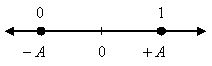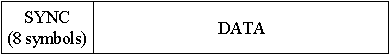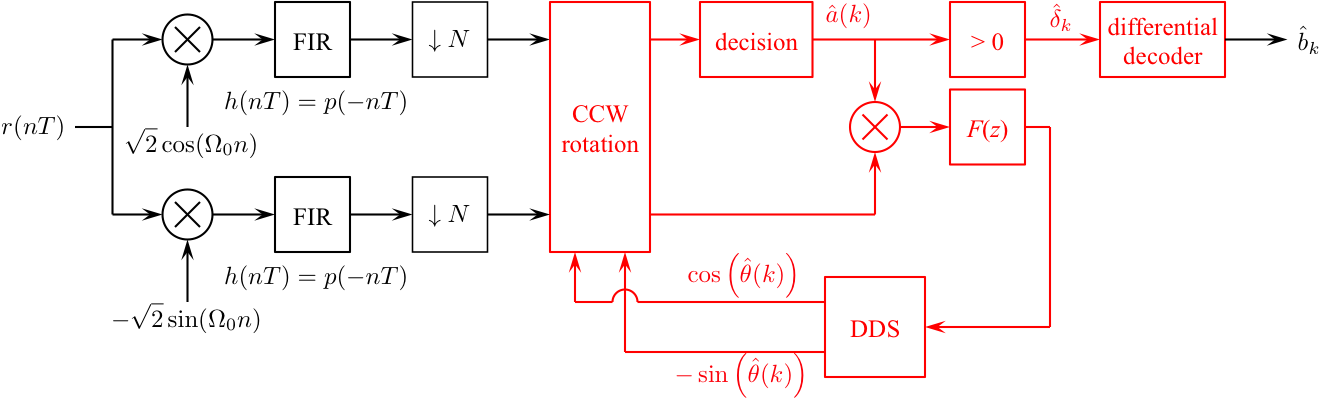# Digital Communications: A Discrete-Time Approachby Michael Rice# Carrier Phase Synchronization for BPSK Using Differential Encoding

## Introduction

In the previous BPSK Simulink Exercise the phase of the unmodulated carrier was assumed known. Unfortunately, this is not the case in practice. The carrier phase is unknown and must be extracted from the received signal using a carrier phase recovery subsystem. In this exercise, you will design a PLL-based carrier phase recovery subsystem for BPSK which will be used to process BPSK modulated data contained in the file bpskcrdata.mat To deal with the 180-degree phase ambiguity of the PLL-based carrier phase synchronizer, this exercise uses differential encoding. In differential encoding, the two possible phase shifts (0 and 180 degrees) are determined by the binary input instead of the absolute phase of the carrier. Detection and phase synchronization are carried out as usual. The resulting decisions, however, are for differentially encoded bits. A differential decoder must be used to recover the information bits.

## Textbook References

M-ary QAM: Section 5.3 (pp. 223 - 240), discrete-time realizations: Section 5.3.2 (pp. 237 - 240), partial response pulse shapes: Section A.2 (pp. 629 - 646), discrete-time PLL: Section C.2 (pp. 380 - 692), carrier phase synchronization for BPSK: Section 7.3 (pp. 348 - 352), differential encoding for phase ambiguity resolution, general discussion: Section 7.7.2 (pp. 368 - 369), BPSK example (pp. 369 - 373).

## Specificationsnormalized sample rate: 8 samples/bit normalized carrier frequency: 0.2 cycles/sample carrier phase: unknown average energy: 1 pulse shape: SRRC (50% excess bandwidth, span = 12 symbols) symbol clock offset: 0 input file bpskcrdedata.mat phase ambiguity resolution differential encoding ```bit phase shift ------------------ 0 180 degrees 1 0 degrees ``` input message length: 280 symbols (280 bits or 40 ASCII characters) packet format SYNC = 0 0 0 1 0 1 1 0 DATA = 280 bits
packet format the data format is shown belowThe packet is repeated 4 times to allow your PLL to acquire and lock.

## Design the Detector

Design the detector, shown below, using blocks from the Simulink, DSP System, and Communications System Toolboxes. I recommend you start with the detector from the BPSK Simulink Exercise and make changes as necessary.Design the loop filter to create a second order loop to the following specifications: closed-loop equivalent noise bandwidth = 0.01 (normalized to the bit rate), damping factor = 0.7071.

## Exercise

1. For the detector input, use the From File block and set the Filename to bpskcrdata.mat and set the sample time to 1.

2. Set the simulation parameters as follows:
 Simulation Time
 Start Time: 0.0 Stop Time: (12+40*7+8)*8*4-1
Solver Options
 Type: Fixed-step Solver: discrete (no continuous states) Fixed step size: auto
Tasking and sample time options
 Periodic sample time constraint: Unconstrained Tasking mode for periodic sample times: SingleTasking

3. Run the simulation.

4. The detector produces 1200 symbol estimates. Even though the SYNC and DATA fields are repeated four times, the PLL does not lock until part way through the sample sequence.

5. To find the data bits, look for the 8-bit SYNC pattern in the detector output. The 280 bits following the SYNC correspond to 40 7-bit ASCII characters.

6. Determine the message using either your Matlab script or an ASCII Table.

7. Plot the phase error (loop filter output) and use this plot to estimate how long (measured in bits) it took your PLL to lock.

8. Plot the eye diagram and signal space projections.
Brigham Young University - Provo | Fulton College of Engineering | The Church of Jesus Christ of Latter-day Saints
Department of Electrical and Computer Engineering, BYU, Provo, UT 84602 - (801)422-4012 - Copyright 2009. All Rights Reserved